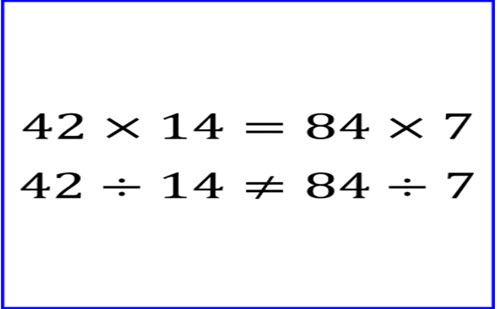Go to website

# Not equal to

Throughout this inquiry students will develop an understanding of division and be introduced to the concept of commutativity. Students learn to recognise the impact that flipping an equation has on the overal outcome. Students work with multiplication and division equations to determine equations which equal the same number, identifying patterns and commonalities.

Year level(s) Year 6
Audience Student, Teacher
Purpose Content knowledge, Student task, Teaching resource, Teaching strategies, Evidence-based approaches
Teaching strategies and pedagogical approaches Collaborative learning, Explicit teaching, Feedback, Growth mindset, Mathematics investigation, Metacognitive strategies, Questioning, Worked examples
Keywords division, multiplication, mental arithmetic, written calculations

## Curriculum alignment

Curriculum connections English, Numeracy, Critical and creative thinking
Strand and focus Number, Apply understanding, Build understanding
Topics Integers, Multiplication and division, Operating with number
AC: Mathematics content descriptions
ACMNA123 Select and apply efficient mental and written strategies and appropriate digital technologies to solve problems involving all four operations with whole numbers
National numeracy learning progression Multiplicative strategies